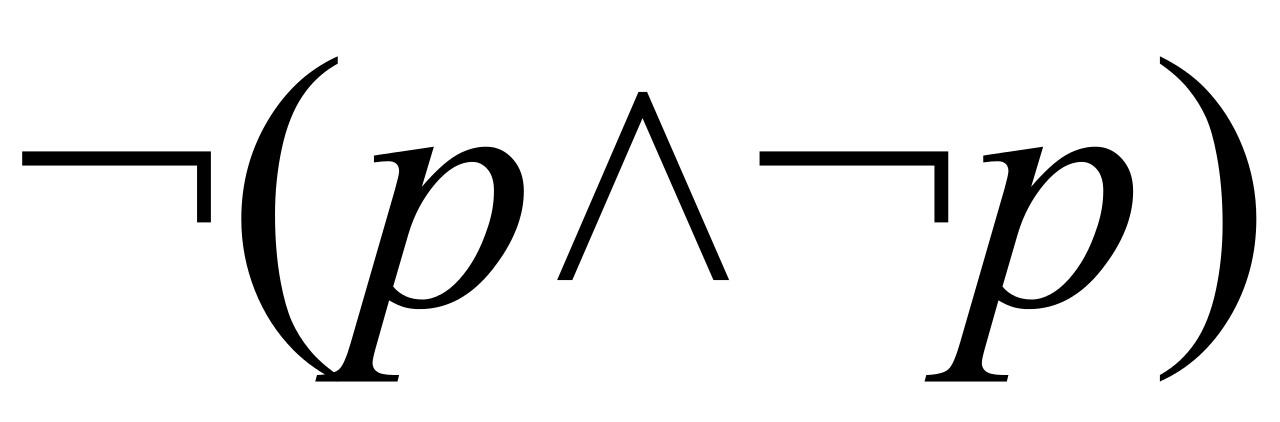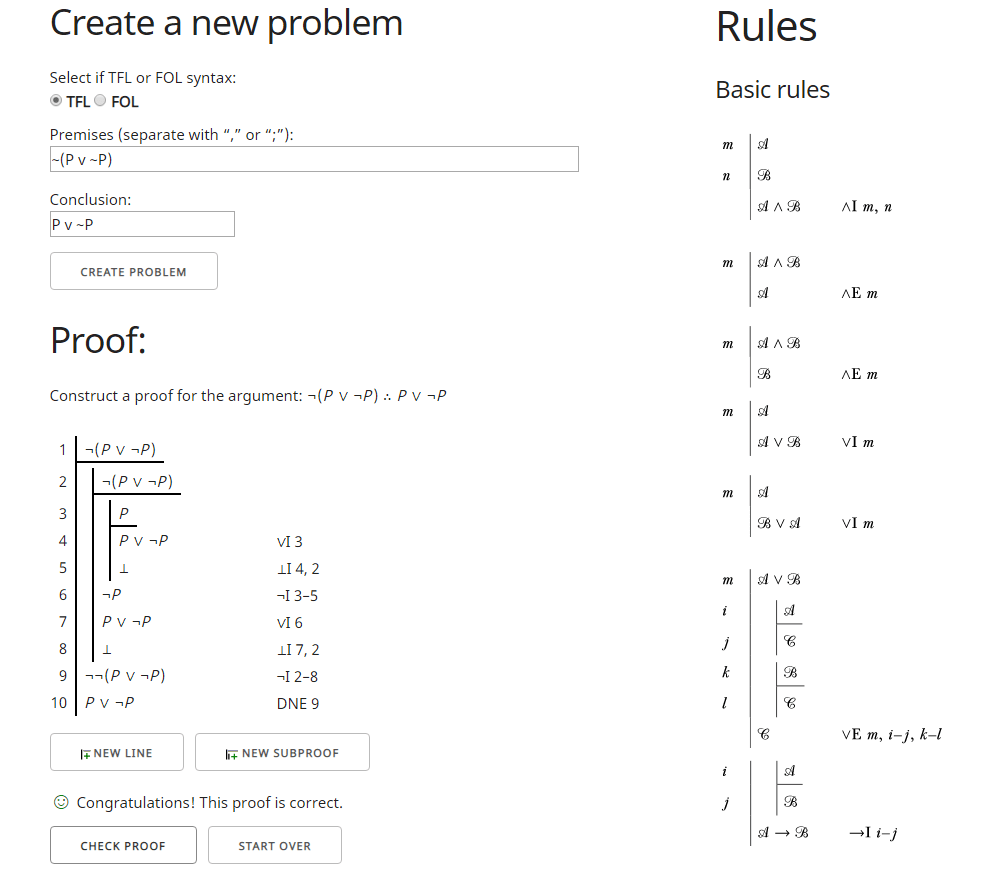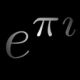# The Basics of the Laws of Logic## The Basics of the Laws of Logic

It is almost an inevitability that in any discussion with a person engaging in presuppositional apologetics that the phrase “the Laws of Logic” will be uttered as some prescriptive way to somehow “prove” or at least validate the existence of God. While they are also known as “laws of thought”, they are really merely descriptive principles of logic or axioms from which classical logic is predicated upon that extend from propositions to ontological states. As analytical propositions they are a priori knowledge which is known by a priori justification independent of experience.

Often these laws of logic can often extend to other forms of logic or semantic interpretations, however, many forms of logic deny or exclude them outright such as paraconsistent logic (a logical system which allows for contradictions without falling into the principle of explosion; ex contradictione quodlibet), dialetheism (a type of paraconsistent logical system which allows for true contradictions), fuzzy logic, intuitionistic logic, and other forms of logical that allow for truth value gluts (a sentence is both T and F) and truth value gaps (a sentence is neither T nor F).

It is generally held that there are 3 traditional, historical, or canonical laws of logic.

The law of Identity:

The Law of Identity is what some consider the most foundational of all the law of logic axioms. Socrates implied it in Plato’s Theaetetus by asking the question “Then do you think that each differs to the other, and is identical to itself?”. Russell more explicitly described it as “Whatever is, is” a shortened version of Parmenides philosophy of whatever is is, while Leibniz referred to it as “Everything is what it is”, and what is not cannot be. Aristotle considered it to be the most fundamental law and obvious truth.

Mathematically the Law of Identity can be represented as:

∀x(x=x)

Which is read as “For all x: x=x” where “=” represents equality and/or identity.

Unlike other laws of logic, the law of identity is related to terms and not propositions, and isn’t used in propositional logic. It more informally can merely be stated as x=x, a=a, or A is A as all relate the same concept of something is itself. Identity is a type of binary relationship which is between the object of equality and itself. This is very closely related to a second order logical principle known to as what Leibniz referred to as identity of indiscernibility:

∀x∀y[∀F(Fx ↔ Fy) x=y]

Read as for “for all of x and y, if x and y have the same properties then x is identical to y” where “Fx” represents the properties of x. (Capital letters tend to represent properties, while lower case represent subjects and referential expressions).

This can also be more explicitly defined by:

x=y =𝒹ₑ𝒻 (∀F)(Fx ↔ Fy)

Where x is the same as y by definition given they have exactly the same properties. Ex: .999… = 1 because “.999…” is just a different type of signifier (an infinite decimal expansion) representing “1” as both have exactly the same properties (they both exist at the same exact point on the real number line and are the same exact value).

#### The law of Non-Contradiction (LNC):

The LNC is that a proposition can not be both true and false at the same time. Propositionally LNC can be defined tautologically as:

LNC =𝒹ₑ𝒻 ¬(P Λ ¬P)

Meaning that given any proposition it can not be both true and false at the same time, or given any two propositions “A is B” and “A is not B” are mutually exclusive. I tend to use, merely by personal choice, capital  “P” or say “A is B” to infer all or any proposition and “p” when referring to a specific proposition…but to the best of my knowledge there is no standard convention on this and ¬(P Λ ~P) and ¬(p Λ ~p) would represent the same thing.

This can also be expressed in terms of metatheory as:

(∀P) ~ (T(P) Λ T(~P))

This would be read as for all propositions it must be the case that the proposition is true or it’s negation is true (as in negation of p is equivalent to p is false).

#### The Law of Excluded Middle (LEM, tertium non datur):

By use of one of DeMorgan’s laws you can derive from the LNC the Law of Excluded Middle, that a proposition must be either true or false:

DeMorgan’s law: ¬(P Λ Q) ↔ (¬P V ¬Q)

Given  ¬(P Λ ~P) you can derive LEM by:

¬(P Λ ¬P)¬P V  ¬ ¬P
¬P V P (double negation rule)*

Propositionally the LEM can then be defined tautologically as:

LEM =𝒹ₑ𝒻  ¬P V P

Or explicitly by law as always true:

P V ¬P ≡ T

*Double negation rule also known as double negation elimination ¬¬P ⇒ P (⇒ means “can be replaced with”), ¬¬P P (Biconditional) or ¬¬P ⊢ P (Sequent notation). In intuitionistic logic double negation rule of A≡ ¬(¬A) does not hold s.t. ~ ¬¬P P at least so far as that p can not be derived directly from double negation.

_______________________

Just for fun I proved you can derive LEM from LNC using a natural logic checker (https://proofs.openlogicproject.org/)  you can also go play around with:1.As mentioned before, I am not a logician, so I have not studied the intricacies of the laws of logic beyond what I needed to write a (usually informal) proof. If I misunderstand, please correct my understanding.

However, I am fascinated by what shifting axioms at the foundations could cause.

Off and on, I’ve been reading a couple books on mathematical logic and axiomatic set theory, from which I hoped to learn more about the foundations. From their exposition, as well as my own experience, mathematics seems to start with a notion of atomic “sentence” or “formula”, something that can be either true or false. Next, the sentential connectives (e.g. disjunction ∨, conjunction ∧) and grouping symbols are introduced to link atomic formulae together into longer structures, subject to certain axioms as to avoid incomplete sentences and other nonsense. The connectives seem to serve as operators, and one can then develop the propositional calculus and basic formal proofs.

From this perspective, I have always seen the Law of Non-Contradiction as a consequence of the binary nature of a proposition: either true or false, but never both. Likewise, the Law of the Excluded Middle arises in a similar way.

That said, I can see how the entire structure would be perturbed if propositions had a third or fourth truth value: “neither” and “both”, or something else. I’d have to know more about how those structures functioned before I could speak to them.

The Law of Identity is one of my particular bugbears. I studied some computer science (CS) in my undergrad career, and I picked up the distinction between definition “:=” and the equality relation “=”, which students and even some of my colleagues blur. Definition, as I understand, is declared to be so by the author, while equality asks whether or not the two objects are identical. In short, “:=” is “because I said so”, and “=” is a consequence of an argument or axiom. I really like the quantification ∀x(x=x) as it reminds me greatly of the reflexive axiom for a partial order or equivalence relation.

On the other hand, I’m a bit wary of Leibniz’s quantification ∀x∀y[∀F(Fx ↔ Fy) → x=y]. This wariness comes from my experience in category theory, where there is a difference between the identity morphism (i.e. isomorphism guaranteed to exist from an object to itself with trivial action under composition) and an arbitrary isomorphism (i.e. morphism with a compositional inverse, defined using the identity morphism). While identical objects are the same, isomorphic objects have the same properties in the given category, i.e. “indiscernible”, as I understand the word.

For example, the natural numbers ℕ:={1,2,3,…} and the integers ℤ:={…,-2,-1,0,1,2,…} are distinct sets, but they are isomorphic in the category of sets as there is a bijective function from one onto the other. They have the same set-theoretic properties, but they are by no means the same set.

Now, viewed in a different category with more structure, ℕ and ℤ are distinct as, say, semirings (or rigs). Equipping both ℕ and ℤ with their usual addition and multiplication, ℤ has an identity and additive inverses, but ℕ does not.

Another example would be the positive real line (0,∞) equipped with multiplication and the entire real line (-∞,∞) equipped with addition, both viewed as groups. The natural exponential and logarithm are group isomorphisms between the two, so their group theoretic properties are identical. However, they certainly are not the same set.

While I’m sure Leibniz’s indiscernibility predates category theory, would one interpret his principle as crossing between categories? That is, tying back to our previous exchange about subsets and universes, what is the domain of discourse for “F” in the quantification “∀x∀y[∀F(Fx ↔ Fy) → x=y]”? Might you have a reference where I can read more on this topic? I’m rather curious about it.

2.1.